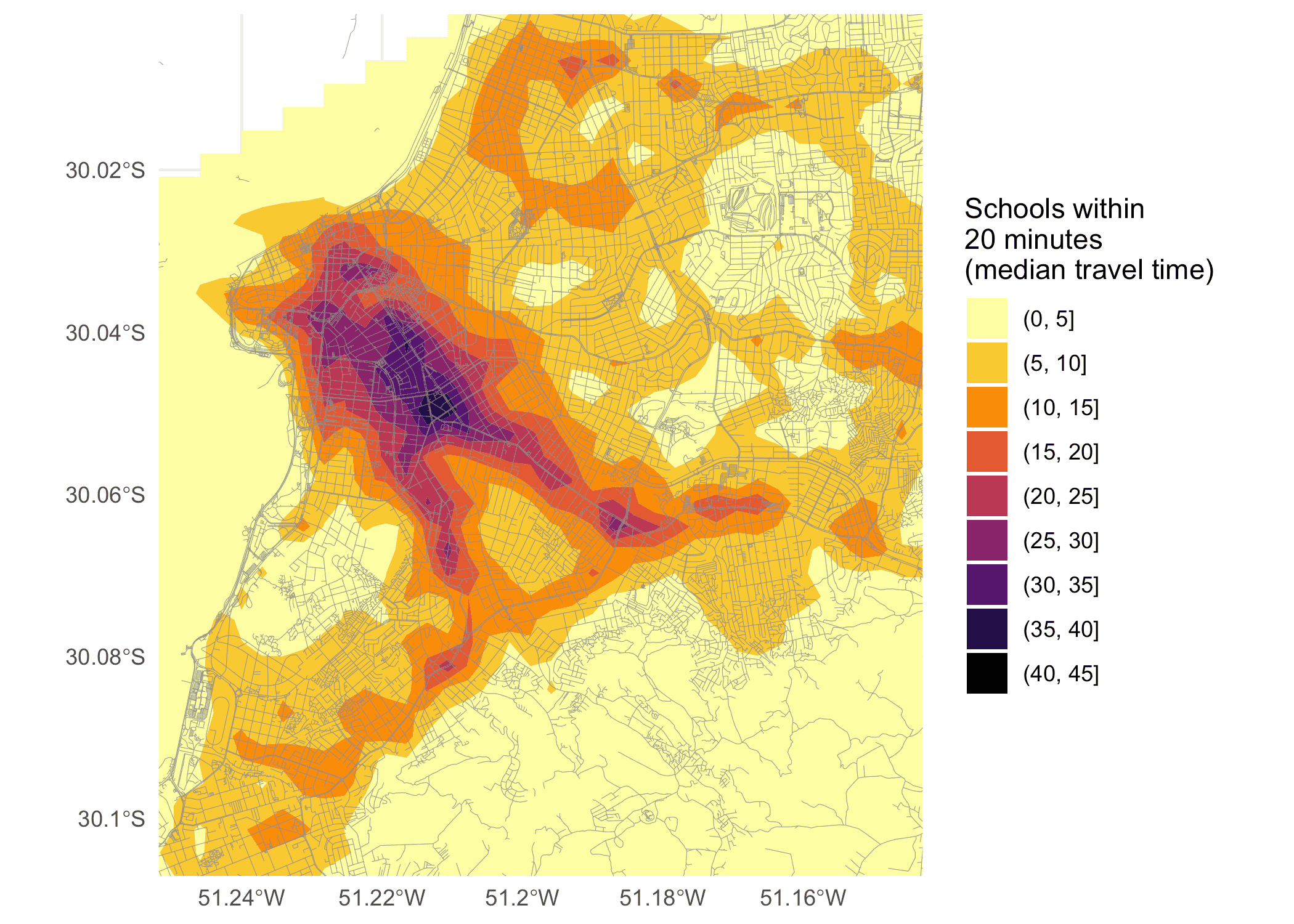Calculating and visualizing Accessibility

2021-10-25

Abstract

This vignette shows how to calculate and visualize accessibility in R using the r5r package.

Introduction

Accessibility metrics measure the ease with which opportunities, such as jobs, can be reached by a traveler from a particular location (Levinson and et al. 2020). One of the simplest forms of accessibility metrics is the cumulative-opportunities, which counts all of the opportunities accessible from each location in less than a cutoff time. Using a travel time matrix and information on the number of opportunities available at each location, we can calculate and map accessibility. This vignette shows how to do that in R using the r5r package.

In this reproducible example, we will be using a sample data set for the city of Porto Alegre (Brazil) included in r5r. We can compute accessibility in 5 quick steps:

1. Increase Java memory and load libraries
2. Build routable transport network
3. Calculate travel times
4. Calculate Accessibility
5. Map Accessibility

Increase Java memory and load libraries

Before we start, we need to increase the memory available to Java and load the packages used in this vignette

options(java.parameters = "-Xmx2G")

library(r5r)
library(sf)
library(data.table)
library(ggplot2)
library(akima)
library(dplyr)

1. Build routable transport network with setup_r5()

To build a routable transport network with r5r and load it into memory, the user needs to call setup_r5 with the path to the directory where OpenStreetMap and GTFS data are stored.

# system.file returns the directory with example data inside the r5r package
# set data path to directory containing your own data if not using the examples
data_path <- system.file("extdata/poa", package = "r5r")

r5r_core <- setup_r5(data_path, verbose = FALSE)

In this example, we will be calculating the number of schools accessible by public transport within a travel time of up to 20 minutes. The sample data provided contains information on the spatial distribution of schools in Porto Alegre in the points$schools. With the code below we compute the median number of schools accessible considering multiple travel time estimates departing every minute over a 60-minute time window, between 2pm and 3pm. # read all points in the city points <- fread(file.path(data_path, "poa_hexgrid.csv")) # routing inputs mode <- c("WALK", "TRANSIT") max_walk_dist <- 1000 # in meters travel_time_cutoff <- 21 # in minutes departure_datetime <- as.POSIXct("13-05-2019 14:00:00", format = "%d-%m-%Y %H:%M:%S") time_window <- 60 # in minutes percentiles <- 50 # calculate travel time matrix access <- accessibility(r5r_core, origins = points, destinations = points, mode = mode, opportunities_colname = "schools", decay_function = "step", cutoffs = travel_time_cutoff, departure_datetime = departure_datetime, max_walk_dist = max_walk_dist, time_window = time_window, percentiles = percentiles, verbose = FALSE) head(access) #> from_id percentile cutoff accessibility #> 1: 89a901291abffff 50 21 3 #> 2: 89a9012a3cfffff 50 21 0 #> 3: 89a901295b7ffff 50 21 6 #> 4: 89a901284a3ffff 50 21 1 #> 5: 89a9012809bffff 50 21 5 #> 6: 89a901285cfffff 50 21 3 3. Map Accessibility The final step is mapping the accessibility results calculated earlier. The code below demonstrates how to do that, with some extra steps to produce a prettier map by doing a spatial interpolation of accessibility estimates. # interpolate estimates to get spatially smooth result access.interp <- access %>% inner_join(points, by=c('from_id'='id')) %>% with(interp(lon, lat, accessibility)) %>% with(cbind(acc=as.vector(z), # Column-major order x=rep(x, times=length(y)), y=rep(y, each=length(x)))) %>% as.data.frame() %>% na.omit() # find results' bounding box to crop the map bb_x <- c(min(access.interp$x), max(access.interp$x)) bb_y <- c(min(access.interp$y), max(access.interp$y)) # extract OSM network, to plot over map street_net <- street_network_to_sf(r5r_core) # plot ggplot(na.omit(access.interp)) + geom_contour_filled(aes(x=x, y=y, z=acc), alpha=.8) + geom_sf(data = street_net$edges, color = "gray55", size=0.1, alpha = 0.9) +
scale_fill_viridis_d(direction = -1, option = 'B') +
scale_x_continuous(expand=c(0,0)) +
scale_y_continuous(expand=c(0,0)) +
coord_sf(xlim = bb_x, ylim = bb_y) +
labs(fill = "Schools within\n20 minutes\n(median travel time)") +
theme_minimal() +
theme(axis.title = element_blank())If you have any suggestions or want to report an error, please visit the package GitHub page.